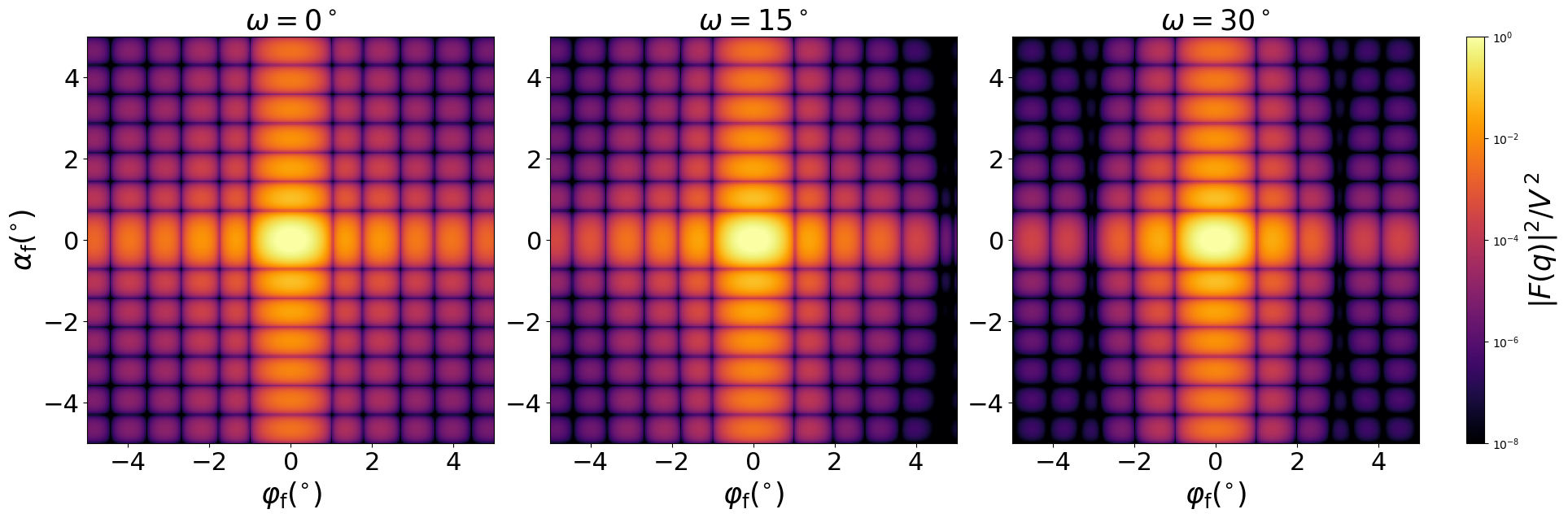### Prism6

A prism based on regular hexagon.#### Constructor

Prism6(L, H)


Parameters:

• R, edge of the regular hexagonal base
• H, height

#### Usage

As for any other Form factor.

#### Implementation

Class Prism6 inherits from the interface class IFormFactor .

Form factor is computed as

$$F(\mathbf{q})=H \space \exp\Big(iq_z\dfrac{H}{2}\Big) \space \text{sinc}\Big(q_z\dfrac{H}{2}\Big) \space F_{||}(\mathbf{q}_{||})$$

where the form factor $F_{||}(\mathbf{q}_{||})$ computation is based on the generic form factor of a planar polygon with two-fold symmetry $(S_2)$ provided by libformfactor .

Volume has been validated against $$V=\dfrac{3\sqrt3}{2}HR^2.$$

More general:

#### Example

Scattering by uncorrelated, oriented prisms for horizontal incidence. Rotation around $z$ axis:Generated by Examples/ff/Prism3.py .

#### History

Has been validated against the “Prism6” form factor of IsGISAXS [manual, Eq. 2.30] and “Prism with six fold symmetry” [Renaud 2009, Eq. 221], which has different parametrization and lacks a factor $H$ in $F(\mathbf{q})$. Reimplemented in BornAgain 1.6 using the generic form factor of a polygonal prism with symmetry $S_{2}$ [Wuttke 2021] to achieve numerical stability near the removable singularity at $q \to 0$.